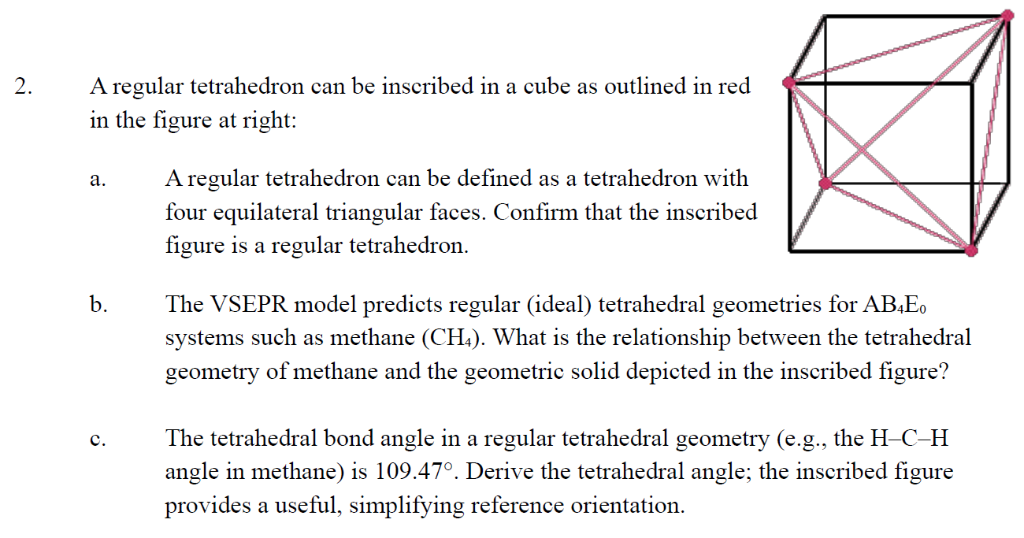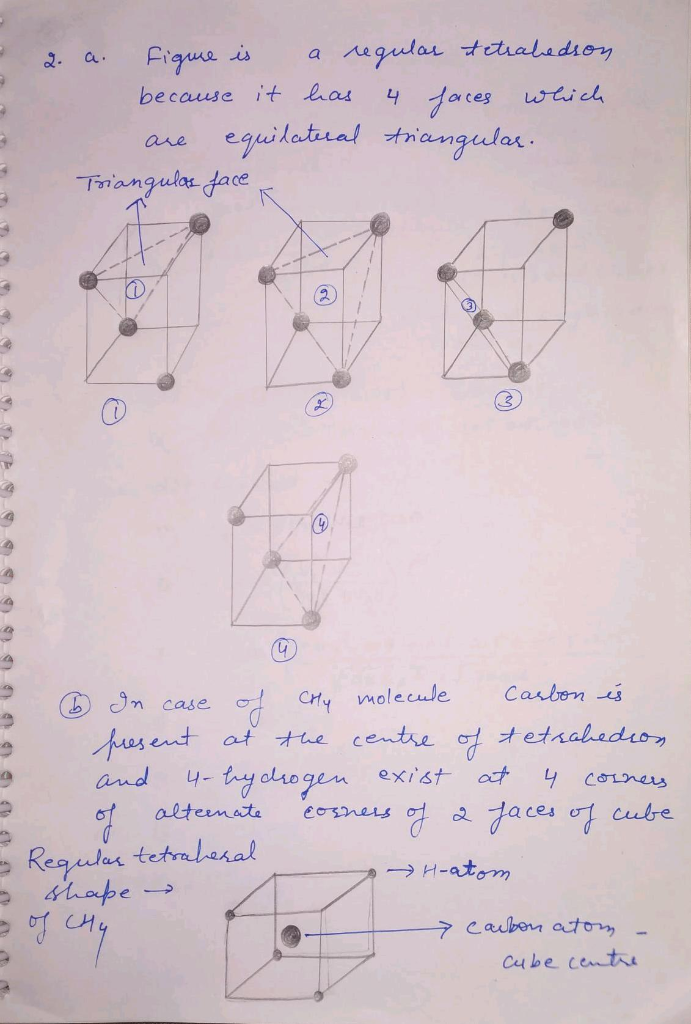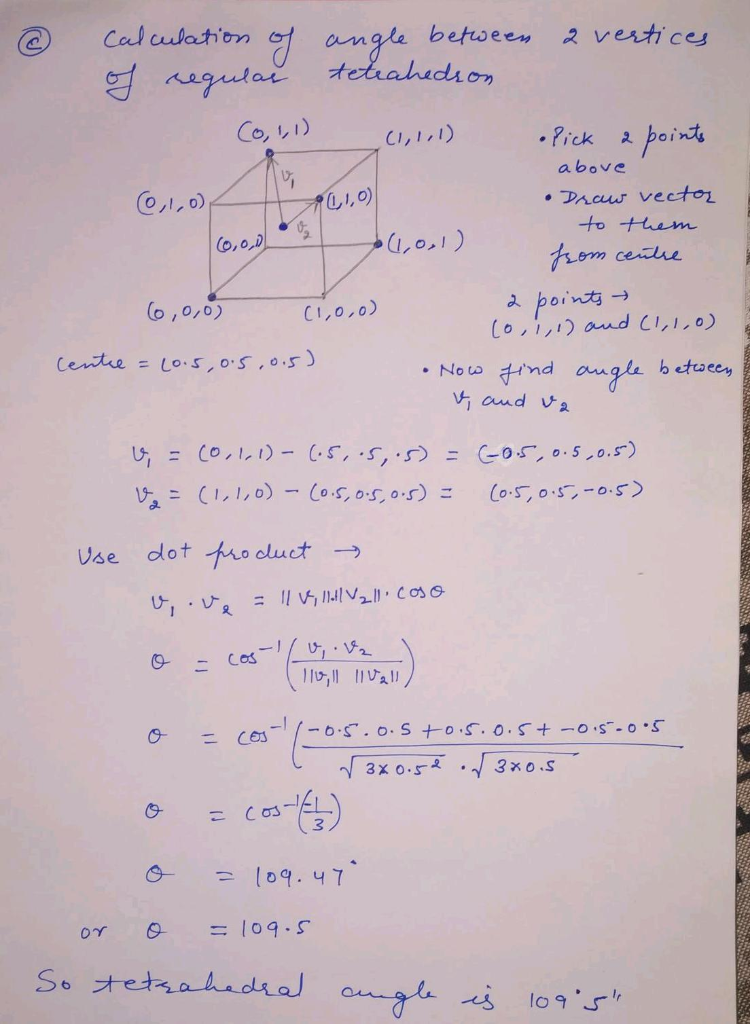# A regular tetrahedron can be inscribed in a cube as outlined in red 2. in the figure right: at A regular tetrahedron ca...A regular tetrahedron can be inscribed in a cube as outlined in red 2. in the figure right: at A regular tetrahedron can be defined as a tetrahedron with a. four equilateral triangular faces. Confirm that the inscribed figure is a regular tetrahedron. The VSEPR model predicts regular (ideal) tetrahedral geometries for AB4E0 systems such as methane (CH4). What is the relationship between the tetrahedral geometry of methane and the geometric solid depicted in the inscribed figure? The tetrahedral bond angle in a regular tetrahedral geometry (e.g., the H-C-H angle in methane) is 109.47°. Derive the tetrahedral angle; the inscribed figure provides a useful, simplifying reference orientation C.